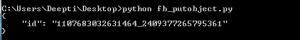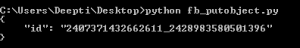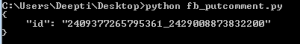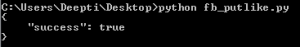Related Articles
• Difficulty Level : Basic
• Last Updated : 26 Jul, 2019

Prerequisites: Facebook API Set-1, Set-2, Set-3

1. put_object
2. put_like
3. put_comment
1. put_object: Writes the mentioned object to the Facebook Social Graph connected to the given parent.
Parameters:

1. parent_object: A string specifying the parent of the new object. For example- Post is the parent of comment, when we want to add a new comment or when we want to add a new photo, User profile is the parent of the photo.
2. connection name: A string specifying the connection or edges between the objects.

Example#1: Post a message with a link to the active user wall.

 `import` `json ` `import` `facebook ` ` `  `def` `main(): ` `    ``token ``=` `"Please replace this with "``me" ``or` `your Access Token(``for` `Posting on your wall)\ ` `             ``or` `with PAGE Access Token(``for` `posting on Page)" ` ` `  `    ``graph ``=` `facebook.GraphAPI(token) ` `    ``message ``=` `graph.put_object(parent_object ``=``"me"``,  ` `              ``connection_name ``=``"feed"``, ` `              ``message ``=``"Hello this is a great website for various Computer Science Topics."``, ` `              ``link ``=``"https://www.geeksforgeeks.com"``) ` ` `  `    ``print``(json.dumps(message, indent ``=` `4``)) ` ` `  `if` `__name__ ``=``=` `'__main__'``: ` `    ``main() ``import` `json ` `import` `facebook ` ` `  `def` `main(): ` `    ``token ``=` `"Please replace this with your PAGE ACCESS TOKEN"` `    ``graph ``=` `facebook.GraphAPI(token) ` `commenttopost ``=` `graph.put_object(parent_object ``=``"PAGEID_POSTID"``, ` `                ``connection_name ``=``"comments"``, ` `                ``message ``=``"Please share and Like the website for content on Computer Science"``) ` `    ``print``(json.dumps(commenttopost, indent ``=` `4``)) ` ` `  `if` `__name__ ``=``=` `'__main__'``: ` `    ``main() `2. put_comment: This methods facilitates to write a message as comment to an object.
Parameters:

1. object_id: A string unique for a particular resource.
2. message: A string to be posted as comment.

 `import` `json ` `import` `facebook ` ` `  `def` `main(): ` `    ``token ``=` `"Please replace this with your PAGE ACCESS TOKEN"` `    ``graph ``=` `facebook.GraphAPI(token) ` `    ``putcomment ``=` `graph.put_comment(object_id ``=``"PAGEID_POSTID"``, ` `                 ``message ``=``"This is a very good website for Computer Science Topics"``) ` ` `  `    ``print``(json.dumps(putcomment, indent ``=` `4``)) ` ` `  `if` `__name__ ``=``=` `'__main__'``: ` `    ``main() `3. put_like: Add like to a given object
Parameters:
object_id: A string that is a unique id for the particular resource.

 `import` `json ` `import` `facebook ` ` `  `def` `main(): ` `    ``token ``=` `"Please replace this with your PAGE ACCESS TOKEN"` `    ``graph ``=` `facebook.GraphAPI(token) ` `    ``putlike ``=` `graph.put_like(object_id ``=``"PAGEID_POSTID"``) ` `    ``print``(json.dumps(putlike, indent ``=` `4``)) ` ` `  `if` `__name__ ``=``=` `'__main__'``: ` `    ``main() `My Personal Notes arrow_drop_up
Recommended Articles
Page :